x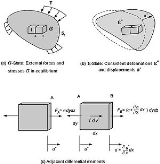Virtual workOverview

Virtual work arises in the application of the principle of least action
Principle of least action
In physics, the principle of least action – or, more accurately, the principle of stationary action – is a variational principle that, when applied to the action of a mechanical system, can be used to obtain the equations of motion for that system...

to the study of forces and movement of a mechanical system
Mechanical system
A mechanical system manages power to accomplish a task that involves forces and movement. Mechanical is derived from the Latin word machina, which in turn derives from the Doric Greek μαχανά , Ionic Greek μηχανή "contrivance, machine, engine" and that from μῆχος , "means, expedient, remedy".The...

. Historically, virtual work and the associated calculus of variations
Calculus of variations
Calculus of variations is a field of mathematics that deals with extremizing functionals, as opposed to ordinary calculus which deals with functions. A functional is usually a mapping from a set of functions to the real numbers. Functionals are often formed as definite integrals involving unknown...

were formulated to analyze systems of rigid bodies, but they have also been developed for the study of the mechanics of deformable bodies.

If a force acts on a particle as it moves from point A to point B, then, for each possible trajectory that the particle may take, it is possible to compute the total work done by the force along the path.Unanswered QuestionsCompaersion between virtual work & other methosAddvantages of virtual work & its limitationEncyclopedia
Virtual work arises in the application of the principle of least action
Principle of least action
In physics, the principle of least action – or, more accurately, the principle of stationary action – is a variational principle that, when applied to the action of a mechanical system, can be used to obtain the equations of motion for that system...

to the study of forces and movement of a mechanical system
Mechanical system
A mechanical system manages power to accomplish a task that involves forces and movement. Mechanical is derived from the Latin word machina, which in turn derives from the Doric Greek μαχανά , Ionic Greek μηχανή "contrivance, machine, engine" and that from μῆχος , "means, expedient, remedy".The...

. Historically, virtual work and the associated calculus of variations
Calculus of variations
Calculus of variations is a field of mathematics that deals with extremizing functionals, as opposed to ordinary calculus which deals with functions. A functional is usually a mapping from a set of functions to the real numbers. Functionals are often formed as definite integrals involving unknown...

were formulated to analyze systems of rigid bodies, but they have also been developed for the study of the mechanics of deformable bodies.

If a force acts on a particle as it moves from point A to point B, then, for each possible trajectory that the particle may take, it is possible to compute the total work done by the force along the path. The principle of virtual work, which is the form of the principle of least action applied to these systems, states that the path actually followed by the particle is the one for which the difference between the work along this path and other nearby paths is zero. The formal procedure for computing the difference of functions evaluated on nearby paths is a generalization of the derivative known from differential calculus, and is termed the calculus of variations.

Let the function x(t) define the path followed by a point, then a nearby path can be defined by adding the function δx(t) to the original path, so the new path is given by x(t)+δx(t). The function δx(t) is called the variation of the original path, and each of the components of δx=(δx, δy, δz) is called a virtual displacement. This can be generalized to an arbitrary mechanical system defined by the generalized coordinates qi, i=1,..., n. In which case, the variation of the trajectory qi(t) is defined by the virtual displacements δqi, i=1,..., n.

Virtual work can now be described as the work done by the applied forces and the inertial forces of a mechanical system as it moves through a set of virtual displacements.

## History

The formulation of virtual work implements the view that nature selects from a set of "tentative" realities in order to minimize a quantity. This is a version of the "simplicity hypothesis" that can be traced to Aristotle. Another form of this hypothesis is Ockham's "razor" which states that "it is futile to employ many principles when it is possible to employ fewer." These ideas illustrate a view of physics that nature optimizes in some way.

Leibnitz formulated Newton's laws of motion in terms of work and kinetic energy, or vis viva (living force), which are minimized as a system moves. Maupertuis adapted Liebnitz's ideas as the principle of least action that nature minimizes action. But it was Euler and Lagrange who provided the mathematical foundation of the calculus of variations and applied it to the study of the statics and dynamics of mechanical systems.

Hamilton's reformulation of the principle of least action and Lagrange's equations yielded a theory of dynamics that is the foundation for modern physics and quantum mechanics.

## Introduction

In this introduction basic definitions are presented that will assist in understanding later sections.

Consider a particle P that moves along a trajectory r(t) from a point A to a point B, while a force F is applied to it, then the work done by the force is given by the integral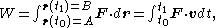where dr is the differential element along the curve that is the trajectory of P, and v is its velocity. It is important to notice that the value of the work W depends on the trajectory r(t).

Now consider the work done by the same force on the same particle P again moving from point A to point B, but this time moving along the nearby trajectory that differs from r(t) by the variation δr(t)=εh(t), where ε is a scaling constant that can be made as small as desired and h(t) is an arbitrary function that satisfies h(t0)=h(t1)=0,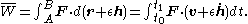The variation of the work δW associated with this nearby path, known as the virtual work, can be computed to be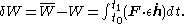Now assume that r(t) and h(t) depend on the generalized coordinates qi, i=1, ..., n, then the derivative of the variation δrh(t) is given by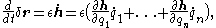then we have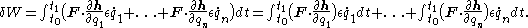The requirement that the virtual work be zero for an arbitrary variation δr(t)=εh(t) is equivalent to the set of requirements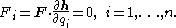The terms Fi are called the generalized forces associated with the virtual displacement δr.

## Static equilibrium

Static equilibrium is the condition in which the applied forces and constraint forces on a mechanical system balance such that the system does not move. The principle of virtual work states that the virtual work of the applied forces is zero for all virtual movements of the system from static equilibrium, that is, δW=0 for any variation δr. This is equivalent to the requirement that the generalized forces for any virtual displacement are zero, that is Fi=0.

Let the forces on the system be Fj, j=1, ..., m and let the virtual displacement of each point of application of these forces be δrj, j=1, ..., m, then the virtual work generated by a virtual displacement of these forces from the equilibrium position is given by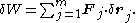Now assume that each δrj depends on the generalized coordinates qi, i=1, ..., n, then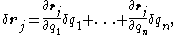and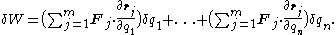The n terms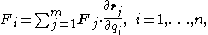are the generalized forces acting on the system. Kane shows that these generalized forces can also be formulated in terms of the ratio of time derivatives,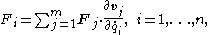where vj is the velocity of the point of application of the force Fj.

In order for the virtual work to be zero for an arbitrary virtual displacement, each of the generalized forces must be zero, that is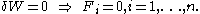### Constraint forces

An important benefit of the principle of virtual work is that only forces that do work as the system moves through a virtual displacement are needed to determine the mechanics of the system. There are many forces in a mechanical system that do no work during a virtual displacement, which means that they need not be considered in this analysis. The two important examples are (i) the internal forces in a rigid body, and (ii) the constraint forces at an ideal joint.

Lanczos presents this as the postulate: "The virtual work of the forces of reaction is always zero for any virtual displacement which is in harmony with the given kinematic constraints." The argument is as follows. The principle of virtual work states in equilibrium the virtual work of the forces applied to a system is zero. Newton's laws state that at equilibrium the applied forces are equal and opposite to the reaction, or constraint, forces. This means the virtual work of the constraint forces must be zero as well.

### Couples

A pair of forces acting on a rigid body can form a couple defined by the moment vector M. The virtual work of a moment vector is obtained from the virtual rotation of the rigid body.

For planar movement, the moment acts perpendicular to the plane with magnitude M and the virtual work due to this moment is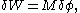where δφ is the virtual rotation angle of the body.

In order to extend this to three dimensional rotations, use the angular velocity vector ω of the body to obtain the virtual work as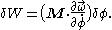Now consider the moments Mj acting on m rigid bodies in a mechanical system. Let the angular velocity vectors ωj, j= 1,..., m of each body depend on the n generalized coordinates qj, i=1,... , n. Then the virtual work obtained from these moments for a virtual displacement from equilibrium is given by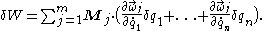Collect the coefficients of the virtual displacements δqi to obtain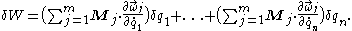### Forces and moments

Combine the virtual work above for couples with the virtual work of forces in order to obtain the virtual work of a system of forces and moments acting on system of rigid bodies displaced from equilibrium as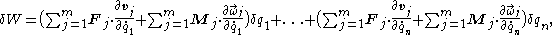where the generalized forces are now defined to be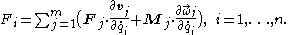The principle of virtual work requires that a system of rigid bodies acted on by the forces and moments Fj and Mj is in equilibrium if the generalized forces Fi are zero, that is## One degree-of-freedom mechanisms

In this section, the principle of virtual work is used for the static analysis of one degree-of-freedom mechanical devices. Specifically, we analyze the lever, a pulley system, a gear train, and a four-bar linkage. Each of these devices moves in the plane, therefore a force F=(fx, fy) has two components and acts on a point with coordinates r= (rx, ry) and velocity v=(vx, vy). A moment, also called a torque
Torque
Torque, moment or moment of force , is the tendency of a force to rotate an object about an axis, fulcrum, or pivot. Just as a force is a push or a pull, a torque can be thought of as a twist....

, T acting on a body that moves in the plane has one component as does the angular velocity ω of the body.

Assume the bodies in the mechanism are rigid and the joints are ideal so that the only change in virtual work is associated with the movement of the input and output forces and torques.

### Applied Forces

Consider a mechanism such as a lever that operates so that an input force generates an output force. Let A be the point where the input force FA is applied, and let B be the point where the output force FB is exerted. Define the position and velocity of A and B by the vectors rA, vA and rB, vB, respectively.

Because the mechanism has one degree-of-freedom, there is a single generalized coordinate q that defines the position vectors rA(q) and rB(q) of the input and output points in the system. The principle of virtual work requires that the generalized force associated with this coordinate be zero, thus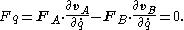The negative sign on the output force FB arises because the convention of virtual work assumes the forces are applied to the device.

### Applied Torque

Consider a mechanism such as a gear train that operates so that an input torque generates an output torque. Let body EA have the input moment TA applied to it, and let body EB exert the output torque TB. Define the angular position and velocity of EA and EB by θA, ωA and θB, ωB, respectively.

Because the mechanism has one degree-of-freedom, there is a single generalized coordinate q that defines the angles θA(q) and θB(q) of the input and output of the system. The principle of virtual work requires that the generalized force associated with this coordinate be zero, thus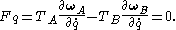The negative sign on the output torque TB arises because the convention of virtual work assumes the torques are applied to the device.

## Law of the Lever

A lever
Lever
In physics, a lever is a rigid object that is used with an appropriate fulcrum or pivot point to either multiply the mechanical force that can be applied to another object or resistance force , or multiply the distance and speed at which the opposite end of the rigid object travels.This leverage...

is modeled as a rigid bar connected a ground frame by a hinged joint called a fulcrum. The lever is operated by applying an input force FA at a point A located by the coordinate vector rA on the bar. The lever then exerts an output force FB at the point B located by rB. The rotation of the lever about the fulcrum P is defined by the rotation angle θ.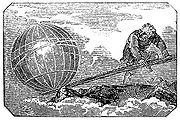Let the coordinate vector of the point P that defines the fulcrum be rP, and introduce the lengths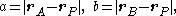which are the distances from the fulcrum to the input point A and to the output point B, respectively.

Now introduce the unit vectors eA and eB from the fulcrum to the point A and B, so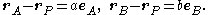This notation allows us to define the velocity of the points A and B as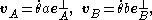where eA and eB are unit vectors perpendicular to eA and eB, respectively.

The angle θ is the generalized coordinate that defines the configuration of the lever, therefore using the formula above for forces applied to a one degree-of-freedom mechanism, the generalized force is given by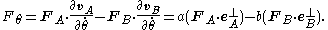Now, denote as FA and FB the components of the forces that are perpendicular to the radial segments PA and PB. These forces are given by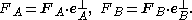This notation and the principle of virtual work yield the formula for the generalized force as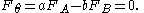The ratio of the output force FB to the input force FA is the mechanical advantage
Mechanical advantage
Mechanical advantage is a measure of the force amplification achieved by using a tool, mechanical device or machine system. Ideally, the device preserves the input power and simply trades off forces against movement to obtain a desired amplification in the output force...

of the lever, and is obtained from the principle of virtual work as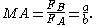This equation shows that if the distance a from the fulcrum to the point A where the input force is applied is greater than the distance b from fulcrum to the point B where the output force is applied, then the lever amplifies the input force. If the opposite is true that the distance from the fulcrum to the input point A is less than from the fulcrum to the output point B, then the lever reduces the magnitude of the input force.

This is the law of the lever, which was proven by Archimedes
Archimedes
Archimedes of Syracuse was a Greek mathematician, physicist, engineer, inventor, and astronomer. Although few details of his life are known, he is regarded as one of the leading scientists in classical antiquity. Among his advances in physics are the foundations of hydrostatics, statics and an...

using geometric reasoning.

## Gear train

A gear train is formed by mounting gears on a frame so that the teeth of the gears engage. Gear teeth are designed to ensure the pitch circles of engaging gears roll on each other without slipping, this provides a smooth transmission of rotation from one gear to the next. For this analysis, we consider a gear train that has one degree-of-freedom, which means the angular rotation of all the gears in the gear train are defined by the angle of the input gear.
The size of the gears and the sequence in which they engage define the ratio of the angular velocity ωA of the input gear to the angular velocity ωB of the output gear, known as the speed ratio, or gear ratio
Gear ratio
The gear ratio of a gear train is the ratio of the angular velocity of the input gear to the angular velocity of the output gear, also known as the speed ratio of the gear train. The gear ratio can be computed directly from the numbers of teeth of the various gears that engage to form the gear...

, of the gear train. Let R be the speed ratio, then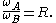The input torque TA acting on the input gear GA is transformed by the gear train into the output torque TB exerted by the output gear GB. If we assume, that the gears are rigid and that there are no losses in the engagement of the gear teeth, then the principle of virtual work can be used to analyze the static equilibrium of the gear train.
Let the angle θ of the input gear be the generalized coordinate of the gear train, then the speed ratio R of the gear train defines the angular velocity of the output gear in terms of the input gear, that is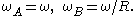The formula above for the principle of virtual work with applied torques yields the generalize force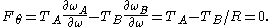The mechanical advantage
Mechanical advantage
Mechanical advantage is a measure of the force amplification achieved by using a tool, mechanical device or machine system. Ideally, the device preserves the input power and simply trades off forces against movement to obtain a desired amplification in the output force...

of the gear train is the ratio of the output torque TB to the input torque TA, and the above equation yields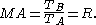Thus, the speed ratio of a gear train also defines its mechanical advantage. This shows that if the input gear rotates faster than the output gear, then the gear train amplifies the input torque. And, if the input gear rotates slower than the output gear, then the gear train reduces the input torque.

## Virtual work principle for a rigid body

If the principle of virtual work for applied forces is used on individual particles of a rigid body
Rigid body
In physics, a rigid body is an idealization of a solid body of finite size in which deformation is neglected. In other words, the distance between any two given points of a rigid body remains constant in time regardless of external forces exerted on it...

, the principle can be generalized for a rigid body: When a rigid body that is in equilibrium is subject to virtual compatible displacements, the total virtual work of all external forces is zero; and conversely, if the total virtual work of all external forces acting on a rigid body is zero then the body is in equilibrium.

The expression compatible displacements means that the particles remain in contact and displace together so that the work done by pairs of action/reaction inter-particle forces cancel out. Various forms of this principle have been credited to Johann (Jean) Bernoulli
Johann Bernoulli
Johann Bernoulli was a Swiss mathematician and was one of the many prominent mathematicians in the Bernoulli family...

(1667-1748) and Daniel Bernoulli
Daniel Bernoulli
Daniel Bernoulli was a Dutch-Swiss mathematician and was one of the many prominent mathematicians in the Bernoulli family. He is particularly remembered for his applications of mathematics to mechanics, especially fluid mechanics, and for his pioneering work in probability and statistics...

(1700-1782).

## Virtual work principle for a deformable body

Consider now the free body diagram
Free body diagram
A free body diagram, also called a force diagram, is a pictorial representation often used by physicists and engineers to analyze the forces acting on a body of interest. A free body diagram shows all forces of all types acting on this body. Drawing such a diagram can aid in solving for the unknown...

of a deformable body, which is composed of an infinite number of differential cubes as shown in the figure. Let's define two unrelated states for the body:
• The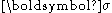-State (Fig.a): This shows external surface forces T, body forces f, and internal stressesin equilibrium.
• The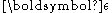-State (Fig.b): This shows continuous displacements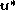and consistent strains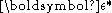.

The superscript * emphasizes that the two states are unrelated. Other than the above stated conditions, there is no need to specify if any of the states are real or virtual.

Imagine now that the forces and stresses in the-State undergo the displacement
Displacement (vector)
A displacement is the shortest distance from the initial to the final position of a point P. Thus, it is the length of an imaginary straight path, typically distinct from the path actually travelled by P...

s and deformations in the-State: We can compute the total virtual (imaginary) work done by all forces acting on the faces of all cubes in two different ways:
• First, by summing the work done by forces such as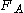which act on individual common faces (Fig.c): Since the material experiences compatible displacement
Displacement (vector)
A displacement is the shortest distance from the initial to the final position of a point P. Thus, it is the length of an imaginary straight path, typically distinct from the path actually travelled by P...

s, such work cancels out, leaving only the virtual work done by the surface forces T (which are equal to stresses on the cubes' faces, by equilibrium).

• Second, by computing the net work done by stresses or forces such as,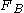which act on an individual cube, e.g. for the one-dimensional case in Fig.(c):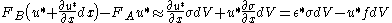where the equilibrium relation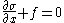has been used and the second order term has been neglected.

Integrating over the whole body gives: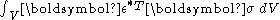- Work done by the body forces f.

Equating the two results leads to the principle of virtual work for a deformable body: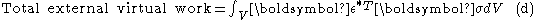where the total external virtual work is done by T and f. Thus,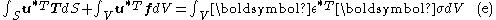The right-hand-side of (d,e) is often called the internal virtual work. The principle of virtual work then states: External virtual work is equal to internal virtual work when equilibrated forces and stresses undergo unrelated but consistent displacements and strains. It includes the principle of virtual work for rigid bodies as a special case where the internal virtual work is zero.

### Proof of Equivalence between the Principle of Virtual Work and the Equilibrium Equation

We start by looking at the total work done by surface traction on the body going through the specified deformation: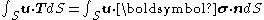Applying divergence theorem to the right hand side yields:Now switch to indicial notation for the ease of derivation.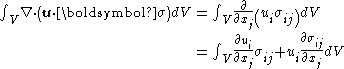To continue our derivation, we substitute in the equilibrium equation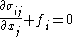. Then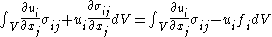The first term on the right hand side needs to be broken into a symmetric part and a skew part as follows: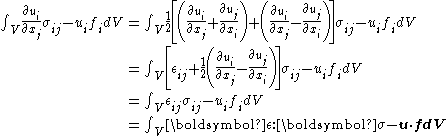whereis the strain that is consistent with the specified displacement field. The 2nd to last equality comes from the fact that the stress matrix is symmetric and that the product of a skew matrix and a symmetric matrix is zero.

Now recap. We have shown through the above derivation that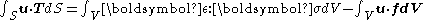Move the 2nd term on the right hand side of the equation to the left: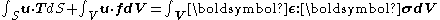The physical interpretation of the above equation is, the External virtual work is equal to internal virtual work when equilibrated forces and stresses undergo unrelated but consistent displacements and strains.

For practical applications:
• In order to impose equilibrium on real stresses and forces, we use consistent virtual displacements and strains in the virtual work equation.

• In order to impose consistent displacements and strains, we use equilibriated virtual stresses and forces in the virtual work equation.

These two general scenarios give rise to two often stated variational principles. They are valid irrespective of material behaviour.

### Principle of virtual displacements

Depending on the purpose, we may specialize the virtual work equation. For example, to derive the principle of virtual displacements in variational notations for supported bodies, we specify:
• Virtual displacements and strains as variations of the real displacements and strains using variational notation such as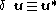and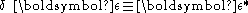• Virtual displacements be zero on the part of the surface that has prescribed displacements, and thus the work done by the reactions is zero. There remains only external surface forces on the part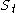that do work.

The virtual work equation then becomes the principle of virtual displacements: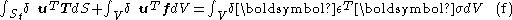This relation is equivalent to the set of equilibrium equations written for a differential element in the deformable body as well as of the stress boundary conditions on the partof the surface. Conversely, (f) can be reached, albeit in a non-trivial manner, by starting with the differential equilibrium equations and the stress boundary conditions on, and proceeding in the manner similar to (a) and (b).

Since virtual displacements are automatically compatible when they are expressed in terms of continuous
Continuous function
In mathematics, a continuous function is a function for which, intuitively, "small" changes in the input result in "small" changes in the output. Otherwise, a function is said to be "discontinuous". A continuous function with a continuous inverse function is called "bicontinuous".Continuity of...

, single-valued function
Single-valued function
A single-valued function is an emphatic term for a mathematical function in the usual sense. That is, each element of the function's domain maps to a single, well-defined element of its range. This contrasts with a general binary relation, which can be viewed as being a multi-valued function...

s, we often mention only the need for consistency between strains and displacements. The virtual work principle is also valid for large real displacements; however, Eq.(f) would then be written using more complex measures of stresses and strains.

### Principle of virtual forces

Here, we specify:
• Virtual forces and stresses as variations of the real forces and stresses.

• Virtual forces be zero on the partof the surface that has prescribed forces, and thus only surface (reaction) forces on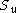(where displacements are prescribed) would do work.

The virtual work equation becomes the principle of virtual forces: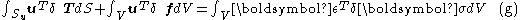This relation is equivalent to the set of strain-compatibility equations as well as of the displacement boundary conditions on the part. It has another name: the principle of complementary virtual work.

## Alternative forms

A specialization of the principle of virtual forces is the unit dummy force method
Unit dummy force method
The Unit dummy force method provides a convenient means for computing displacements in structural systems. It is applicable for both linear and non-linear material behaviours as well as for systems subject to environmental effects, and hence more general than Castigliano's second theorem.-Discrete...

, which is very useful for computing displacements in structural systems. According to D'Alembert's principle
D'Alembert's principle
D'Alembert's principle, also known as the Lagrange–d'Alembert principle, is a statement of the fundamental classical laws of motion. It is named after its discoverer, the French physicist and mathematician Jean le Rond d'Alembert...

, inclusion of inertial forces as additional body forces will give the virtual work equation applicable to dynamical systems. More generalized principles can be derived by:
• allowing variations of all quantities.
• using Lagrange multipliers
Lagrange multipliers
In mathematical optimization, the method of Lagrange multipliers provides a strategy for finding the maxima and minima of a function subject to constraints.For instance , consider the optimization problem...

to impose boundary conditions and/or to relax the conditions specified in the two states.

These are described in some of the references.

Among the many energy principles in structural mechanics
Energy principles in structural mechanics
Energy principles in structural mechanics express the relationships between stresses, strains or deformations, displacements, material properties, and external effects in the form of energy or work done by internal and external forces...

, the virtual work principle deserves a special place due to its generality that leads to powerful applications in structural analysis
Structural analysis
Structural analysis is the determination of the effects of loads on physical structures and their components. Structures subject to this type of analysis include all that must withstand loads, such as buildings, bridges, vehicles, machinery, furniture, attire, soil strata, prostheses and...

, solid mechanics
Solid mechanics
Solid mechanics is the branch of mechanics, physics, and mathematics that concerns the behavior of solid matter under external actions . It is part of a broader study known as continuum mechanics. One of the most common practical applications of solid mechanics is the Euler-Bernoulli beam equation...

, and finite element method in structural mechanics
Finite element method in structural mechanics
The Finite element method is a powerful technique originally developed for numerical solution of complex problems in structural mechanics, and it remains the method of choice for complex systems. In the FEM, the structural system is modeled by a set of appropriate finite elements interconnected at...

.

## See also

• Flexibility method
Flexibility method
In structural engineering, the flexibility method is the classical consistent deformation method for computing member forces and displacements in structural systems...

• Unit dummy force method
Unit dummy force method
The Unit dummy force method provides a convenient means for computing displacements in structural systems. It is applicable for both linear and non-linear material behaviours as well as for systems subject to environmental effects, and hence more general than Castigliano's second theorem.-Discrete...

• Finite element method in structural mechanics
Finite element method in structural mechanics
The Finite element method is a powerful technique originally developed for numerical solution of complex problems in structural mechanics, and it remains the method of choice for complex systems. In the FEM, the structural system is modeled by a set of appropriate finite elements interconnected at...

• Calculus of variations
Calculus of variations
Calculus of variations is a field of mathematics that deals with extremizing functionals, as opposed to ordinary calculus which deals with functions. A functional is usually a mapping from a set of functions to the real numbers. Functionals are often formed as definite integrals involving unknown...

• Lagrangian mechanics
Lagrangian mechanics
Lagrangian mechanics is a re-formulation of classical mechanics that combines conservation of momentum with conservation of energy. It was introduced by the Italian-French mathematician Joseph-Louis Lagrange in 1788....

The source of this article is wikipedia, the free encyclopedia.  The text of this article is licensed under the GFDL.